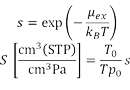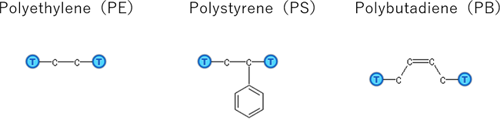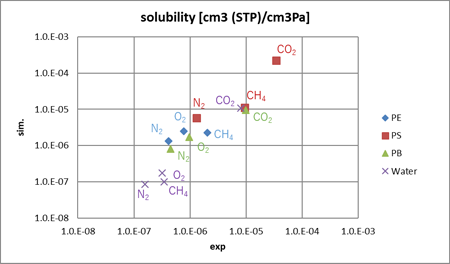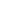Other methods
Small molecule penetration / diffusion / adsorption
Materials Science

# Calculation of solubility coefficient

J-OCTA's solubility module calculates the solubility coefficient from the value of excess chemical potential.

## Purpose and method

The solubility coefficient is an important factor in evaluating permeability of a polymer. J-OCTA's solubility module can calculate the excess chemical potential μex and the solubility coefficient S when gas molecules are dissolved in the polymer. When calculating the excess chemical potential, the Excluded Volume Map Sampling (EVMS) method  is utilized for sampling efficiency in the module.s: Dimensionless solubility called Henry's constant

kB: Boltzmann's constant

T: Temperature of the polymer system

T0: Temperature of the standard condition

p0: Pressure of the standard condition

In this case study, we have calculated the solubility coefficients of the gas molecules (N2, O2, CO2, and CH4) for three polymers (Figure 1) and water. The polymers had 50 monomer units, and GAFF was used for the force field. Dreiding force field was used for the gas molecules, and SPC-FW model was used for the water model. The polymers were relaxed by MD simulation for 3ns with NPT ensemble, then solubility coefficients were evaluated.Figure 1. Polymers for this calculation

## Simulation result

We calculated the solubility coefficients of the gas molecules in the three polymers and water. The results are shown in Figure 2.
Among the polymers, the solubility in PS tends to be higher. Among the gas molecules, the non-polar N2 and O2 molecules tend to have low solubility and CO2 molecule tends to have high solubility, and this shows a good agreement between simulations and experiments.Figure 2 Comparison of calculated solubility coefficients with experimental values
Experimental values for PE, PS, PB, and Water are taken from the literature [2-5], respectively.

• Reference
•  G. L. Deitrick,L.E.Scriven, and H.T.Davis, J. Chem. Phys., 90,2370 (1989)
•  A. S. Michaels and H. J. Bixler, J. Polym. Sci., vol. 50, no. 154, pp. 393–412, 1961.
•  W. R. Vieth, P. M. Tam, and A. S. Michaels, J. Colloid Interface Sci., vol. 22, no. 4, pp. 360–370, 1966.
•  Polymer Handbook
•  R. Sander, Atmos. Chem. Phys., vol. 15, no. 8, pp. 4399–4981, 2015.This website uses cookies to improve functionality and performance. If you continue browsing the site, you are giving implied consent to the use of cookies on this website. If you want to know more or refuse consent, read our Cookie Policy.

Accept